Home / Basic Electrical / RLC Resonance, Acceptor, Tank & Rejector Circuit

# RLC Resonance, Acceptor, Tank & Rejector Circuit

Want create site? Find Free WordPress Themes and plugins.

RLC networks are ac circuits that have resistors, capacitors, and inductors placed in the circuit to pass, reject, or control current. A circuit containing all three factors—resistance, inductance, and capacitance—is called an RLC circuit.

The resulting impedance in an RLC circuit is equal to the vector addition of R in ohms, XL in ohms, and XC in ohms.

The principles of resistance, capacitance, and inductance should be reviewed before studying these combination circuits.

• In an ac circuit containing resistance only, the applied voltage and current are in phase. There is no reactive power. The power consumed by the circuit is equal to the product of volts time’s amperes.
• In an ac circuit containing inductance only, the current lags the voltage by an angle of 90 degrees. They are not in phase. The power consumed by the circuit is zero.
• In an ac circuit containing resistance and inductance, the current lags the voltage by a phase angle of less than 90 degrees. The total resistive force is the vector sum of the resistance and the inductive reactance. This is the impedance of the circuit.
• In an ac circuit containing capacitance only, the current leads the voltage by an angle of 90 degrees. The power consumed is zero.
• In an ac circuit containing resistance and capacitance, the current leads the voltage by an angle of less than 90 degrees. The impedance is equal to the vector sum of the resistance and the capacitive reactance. This is the impedance of the circuit.

## RLC Resonance Circuit

A special condition exists in an RLC circuit when it is energized at a frequency at which the inductive reactance is equal to the capacitive reactance (XL = XC).

Since XL increases as frequency increases and XC decreases as frequency increases, there is one frequency at which both reactances are equal. This frequency is called the resonant frequency of the circuit, or fo.

A series or parallel RLC circuit at the resonant frequency is known as a tuned circuit.

In the vector diagram, Figure 1, XL equals 100 Ω, XC equals 100 Ω, and R equals 50 Ω. XL and XC are opposing each other because they are 180 degrees out of phase.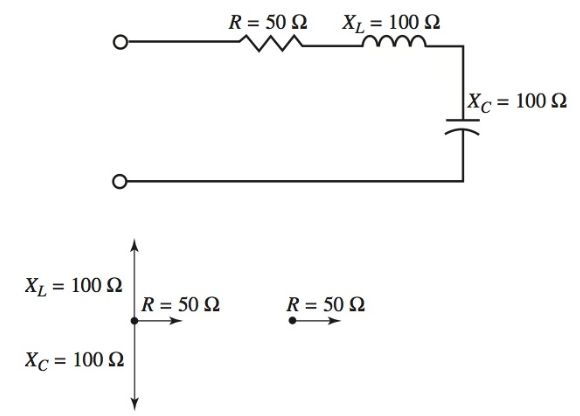Figure 1. Resonance exists when XL = XC and the circuit appears as a resistive circuit.

The algebraic sum of these vectors is zero, so only a resistance of 50 ohms remains. The current and voltage are in phase. This particular circuit frequency can be calculated using the resonant frequency formula. This formula is stated:

${{f}_{o}}=\frac{1}{2\pi \sqrt{LC}}=\frac{0.159}{\sqrt{LC}}$

Where fo equals resonant frequency in hertz, L equals inductance in henrys, and C equals capacitance in farads. This formula is arrived at using the following steps.

At resonance, XL = XC, or:

$2\pi fL=\frac{1}{2\pi fC}$

Move the 2πL to the right side of the equation by dividing both sides by 2πL. This leaves you with:

$f=\frac{1}{{{\left( 2\pi \right)}^{2}}fLC}$

Move the f in the denominator of the right side of the equation to the left side by multiplying both sides by f.

${{f}^{2}}=\frac{1}{{{\left( 2\pi \right)}^{2}}LC}$

Take square root of both sides of the equation.

$f=\frac{1}{2\pi \sqrt{LC}}$

The equation can be simplified further by:

$\frac{1}{2\pi }\cong 0.159$

Therefore:

${{f}_{o}}=\frac{0.159}{\sqrt{LC}}\left( approximately \right)$

RLC Resonant Frequency Calculation Example 1

What is the resonant frequency of a circuit that has 200 μH inductance and 200 pF capacitance?

\begin{align} & {{f}_{o}}=\frac{0.159}{\sqrt{LC}}=\frac{0.159}{\sqrt{200*{{10}^{-6}}*200*{{10}^{-12}}}}=0.08*{{10}^{7}}=800,000Hz \\ & {{f}_{o}}=800kHz\text{ }or\text{ }0.8MHz \\\end{align}

In electronic work, L and C usually have very small values. They are usually in the milli, micro, nano, and Pico ranges. By restating the equation, you can create a shortcut for yourself. The equation can be stated as:

${{f}_{o}}=\frac{0.159}{\sqrt{LC}}$

Where fo equals resonant frequency in megahertz, L equals inductance in millihenrys, and C equals capacitance in nanoFarads.

Or

Where fo equals resonant frequency in megahertz, L equals inductance in microhenrys, and C equals capacitance in microfarads.

## RLC Acceptor Circuit

A series RLC circuit is drawn in Figure 2. At resonance XL = XC, so the impedance of the circuit equals:

$Z=\sqrt{{{R}^{2}}+{{\left( {{X}_{L}}-{{X}_{C}} \right)}^{2}}}=\sqrt{{{R}^{2}}}=R$Figure 2. The series resonant circuit is an acceptor circuit.

Only the ohmic resistance impedes the current in the circuit. In a series resonant circuit, the impedance is at a minimum.

At frequencies above or below the resonant frequency, XL is not equal to XC and the reactive component increases the impedance of the circuit.

The response of a series tuned circuit appears as a bell-shaped curve, Figure 3. Notice, on the graph, that the impedance of the circuit is minimum at resonance.

Note also that maximum current flows at resonance. The current rapidly falls off on either side of the resonant frequency due to the increased impedance, Figure 4. This circuit is called an acceptor circuit.

An acceptor circuit provides maximum response to currents at its resonant frequency.Figure 3. The curve shows the increase in impedance as the frequency is varied above or below resonance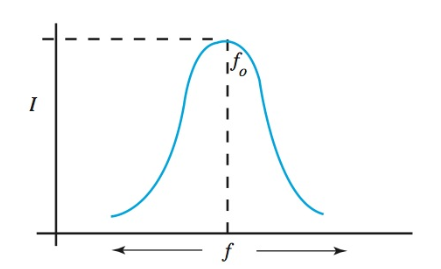Figure 4. The response curve showing the falling off the current in the circuit above or below resonance.

## RLC Acceptor Circuit Example

Refer to Figure 5. This circuit has 10 Ω of resistance, 200 μH of inductance, and 200 pF of capacitance. These components are connected across a 500 μV radio frequency generator at 800 kilohertz. The resonant frequency of this RLC circuit is 800 kilohertz, so XL equals XC at 800 kHz. Z equals 10 ohms at this frequency and:

$I=\frac{E}{Z}=\frac{500*{{10}^{-6}}V}{10\Omega }=50\mu A$

Also:

${{X}_{L}}=2\pi fL=2\pi *800*{{10}^{3}}Hz*200*{{10}^{-6}}H=1000\Omega$

And:

${{X}_{L}}=\frac{1}{2\pi fC}=\frac{1}{2\pi *800*{{10}^{3}}Hz*200*{{10}^{-12}}H}=1000\Omega$

The voltage drops around the circuit equal:

\begin{align} & {{E}_{R}}=50\mu A*10\Omega =500\mu V \\ & {{E}_{{{X}_{L}}}}=50\mu A*1000\Omega =50,000\mu V \\ & {{E}_{{{X}_{C}}}}=50\mu A*1000\Omega =50,000\mu V \\\end{align}

It appears that the sum of these voltages would be 100,500 μV. However, EXL and EXC are 180 degrees out of phase. Therefore, a vector addition must be made:

\begin{align} & {{E}_{source}}=\sqrt{{{\left( {{E}_{R}} \right)}^{2}}+{{\left( {{E}_{{{X}_{L}}}}-{{E}_{{{X}_{C}}}} \right)}^{2}}} \\ & =\sqrt{{{\left( 500\mu V \right)}^{2}}+{{\left( 50,000\mu V-50,000\mu V \right)}^{2}}} \\ & =500\mu V \\\end{align}

Note that, at resonance, the voltage drops across XL and XC are equal. Also, the voltage drop across R equals the source voltage.Figure 5. A series tuned acceptor circuit.

In a resonant circuit, an interchange of energy between the inductance and the capacitance builds up voltage that exceeds the supply voltage.

In the first half cycle, the magnetic field of the inductor stores the energy of the discharging capacitor. In the next half cycle, the stored energy in the magnetic field of the inductor charges the capacitor. This action occurs back and forth, limited only by the series resistance between the two components.

At resonance, the charging times for the inductor and capacitance must be the same, and they will have a canceling effect.

Summarizing the series tuned circuit:

• At resonance, the impedance is a minimum and the line current is a maximum.
• At resonance, the voltage drop, EXL, is equal to EXC, but is 180 degrees out of phase.
• The vector sum of all voltage drops equals the applied voltage. Figure 6 shows how values change as circuit frequency changes.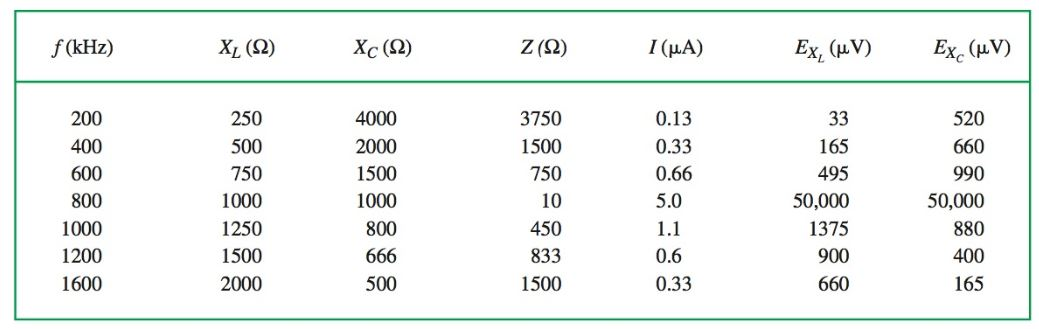Figure 6. As circuit frequency changes, other values also change. The figures used are rounded to the nearest whole number to ease understanding. The resistance of 10 ohms is insignificant. It is not included in computations, except at resonance.

## RLC Tank Circuit

Study Figure 7. It shows a parallel tuned circuit. This type of tuned circuit behaves very differently from a series tuned acceptor circuit.

In part A of Figure 7, Switch 1 is closed and capacitor C is charged to the supply voltage. When Switch 1 is opened, C remains charged.

When Switch 2 is closed (part B), the capacitor discharges through L in the direction of the arrows shown. As the current flows through L, a magnetic field builds around L. This field remains as long as the current flows.

When the charges on the plates of C become equalized, current ceases to flow. The magnetic field around L then collapses. The energy stored in this field is returned to the circuit.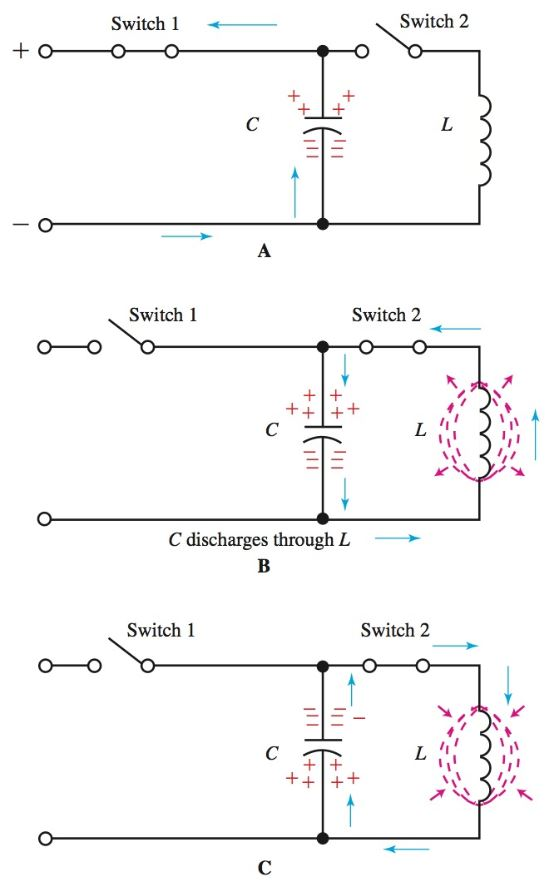Figure 7. The charge and discharge of C through inductance L is similar to flywheel action. It is called a tank circuit.

A–Capacitor charges.

B–Capacitor discharges.

C–The field around the inductor collapses, which charges the capacitor to the opposite polarity.

We know that the induced emf opposes the current change. This is also true in the parallel RLC circuit.

When the current drops to zero as a result of the discharged capacitor, a current is induced by the collapsing magnetic field. This current then drives a charge onto the capacitor, but opposite to the original polarity (part C).

Later the capacitor discharges in the opposite direction. Once again the same cycle of events occurs. The capacitor again becomes charged as in its original state. This discharge/charge cycle repeats over and over. The current periodically changes direction in the circuit. A circuit containing this periodic changing current is said to be oscillating.

The periodic current changes in the circuit can be described as flywheel action. This parallel RLC circuit is called a tank circuit. If no energy were used during the cycles of oscillation, the circuit might oscillate indefinitely. But there is always some resistance due to coil windings and circuit connections. This resistance uses up the energy stored in the circuit and dampens out the oscillation.

The amplitude, or size, of each successive oscillation, decreases due to the resistance.

Compare the oscillators of a tank circuit to a child on a swing. The child can be swinging back and forth, or oscillating, but if no one adds a little push to the swing, the amplitude of the swing will decrease until it comes to rest. If not for friction or air resistance, the swing might continue swinging back and forth forever.

Now consider what would happen if the swing was pushed every time it reached its maximum backward position. This added energy would replace the energy lost to friction. The full swinging action would continue.

A tank circuit is similar to this swing example. If pulses of energy are added to the oscillating tank circuit at the correct frequency, it will continue to oscillate.

What is the meaning of correct frequency? During the discharge and charge of the capacitor C in Figure 7, a set amount of time must pass.

In other words, during one cycle of oscillation, a set interval of time must pass. The number of cycles that occur in one second would be called the frequency of oscillation.

Frequency is measured in cycles per second (cps) or Hertz (Hz).

Close study of this circuit reveals that if C or L were made larger (so that they require a longer time to charge) the frequency of the circuit decreases. This relationship can be shown using the resonance formula:

${{f}_{o}}=\frac{1}{2\pi \sqrt{LC}}$

Notice that both L and C are inversely proportional to the fo. If L or C is made large, fo will become proportionally smaller.

## RLC Rejector Circuit

Figure 8 shows a parallel tuned circuit connected across a variable frequency generator. Minimum line current flows at the resonant frequency of this tuned circuit.

The resonant frequency can be found by observing the minimum value in the line current. This minimum value shows up as a dip that can be measured by a current meter in the line.

Radio transmitter operators always “dip the final.” This term means that the final tank circuit is tuned to resonance, which is indicated by a dip in current in the final circuit.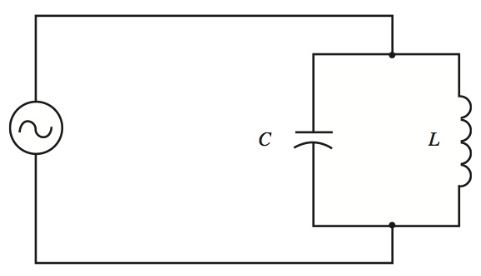Figure 8. A parallel LC circuit connected to a variable frequency generator.

Since line current in the circuit at resonance is a minimum, a parallel tuned circuit has a maximum line impedance (Z).

At frequencies other than resonance, the impedance is much less. So, the parallel tuned circuit rejects signals at or near its resonant frequency and allows signals of frequencies other than resonance to pass. This is why the parallel tuned circuit is called a rejector circuit. These characteristics are shown in the response curves in Figure 9.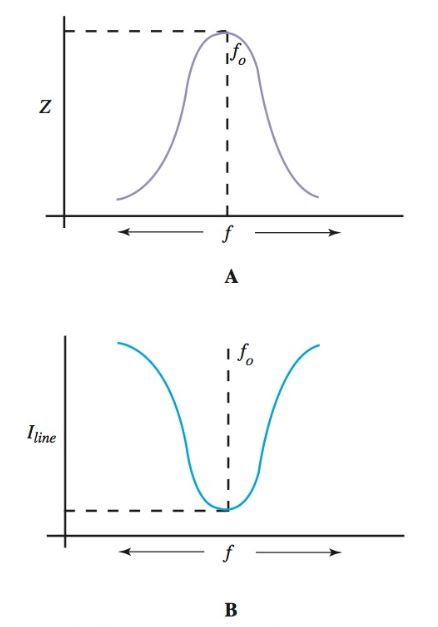Figure 9. A–Curve shows maximum impedance at the resonant frequency. B–Circuit response shows a minimum line current at resonance.

Why does a parallel tuned tank present maximum impedance at resonance?

At resonance XL = XC. Both paths have reactive values in parallel across the generator source. It would appear that these two reactive branches would combine to form a low reactive path for the line current. However, the current flowing in the XL branch lags the applied voltage by 90 degrees. The current in the XC branch leads the applied voltage by 90 degrees. The currents, therefore, are 180 degrees out of phase and cancel each other.

The total line current is the sum of the branch currents. It is zero, except for a small amount of current that flows due to the resistance of the wire in the coil.

## Parallel Resonant Circuit Example

Refer to Figure 10. A 200 μH inductor and a 200 pF capacitor are connected in parallel across a generator source of 500 μV. The resistance, 10 ohms, represents the lumped resistance of the wire of the inductor. These same components were used in the study of the series resonant circuit. Compare the results of the two circuits at resonance.Figure 10. This parallel resonant circuit has a 200 μH inductor and a 200 pF capacitor. They are connected in parallel across a 500 μV generator source.

The resonant frequency of this tuned circuit is:

${{f}_{o}}=\frac{0.159}{\sqrt{LC}}=\frac{0.159}{\sqrt{200\mu H*\left( 0.2*10-3 \right)\mu F}}=0.8MHz$

(Note use of convenient formula when L is in μH, C is in μF, and fo is in megahertz.) At resonance:

${{X}_{L}}=2\pi fL=2\pi *800*{{10}^{3}}Hz*200*{{10}^{-6}}H=1000\Omega$

And:

${{X}_{L}}=\frac{1}{2\pi fC}=\frac{1}{2\pi *800*{{10}^{3}}Hz*200*{{10}^{-12}}H}=1000\Omega$

The voltage across both branches of the parallel circuit is the same as the applied voltage, or 500 μV. Therefore, the current in the XL and XC branch would be:

\begin{align} & {{I}_{{{X}_{L}}}}=\frac{{{E}_{L}}}{{{X}_{L}}}=\frac{500\mu V}{1000\Omega }=0.5\mu A \\ & {{I}_{{{X}_{C}}}}=\frac{{{E}_{C}}}{{{X}_{C}}}=\frac{500\mu V}{1000\Omega }=0.5\mu A \\\end{align}

Since these two currents are 180 degrees out of phase:

${{I}_{{{X}_{L}}}}=\text{ }{{I}_{{{X}_{C}}}}=\text{ }0.5\mu A-0.5\mu A=0$

In this exercise, R has been overlooked because of its small value. However, some current will flow as a result of R. For frequencies above and below resonance, the action of the circuit can be seen in Figure 11.Figure 11. Circuit performance for frequency above and below resonance.

Note that the line current is the difference between the branch currents. Figure 11 could be carried a step further to show the decreasing impedance due to frequencies other than resonance for:

$Z=\frac{E}{I}$

As Z decreases, I must increase.

## Q of Tuned Circuits

The Q, figure of merit, or quality factor, of the circuit, is the link between inductive reactance and resistance in the circuit. Q has no units. The Q of a circuit can be found using the formula:

$Q=\frac{{{X}_{L}}}{R}$

Where Q is the quality factor, XL is the inductive reactance at resonance, and R is the resistance.

Example: In a series circuit, the inductive reactance is 1000 ohms at resonance, and the resistance of the wire of the coil is 10 ohms. The figure of merit, Q, would be:

$Q=\frac{{{X}_{L}}}{R}=\frac{1000\Omega }{10\Omega }=100$

## Acceptor Circuit Q

The Q of a circuit indicates the sharpness of the reject or accept characteristics of the parallel or series RLC circuit. It is a quality factor. In the series acceptor circuit, an increase in resistance reduces the maximum current at resonance, Figure 12. The Q can also be used to determine the rise in voltage across L or C at resonance.

${{E}_{{{X}_{L}}}}={{E}_{{{X}_{C}}}}=Q\times {{E}_{S}}$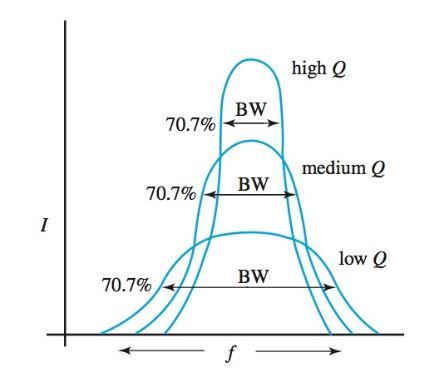Figure 12. As the Q of a circuit is lowered, the curve flattens out. Its selectivity decreases and its bandwidth increases.

Refer back to Figure 5. The supply voltage is 500 μV and the Q of this circuit is 100. Therefore, the voltage rise across XL or XC at resonance is equal to:

${{E}_{{{X}_{L}}}}={{E}_{{{X}_{C}}}}=Q\times {{E}_{S}}=100*500\mu V=50,000\mu V$

If the circuit had a lower Q, the magnified voltage at resonance would be much less. For example, increase R to 20 ohms. Now, the Q would equal only 50. The voltage rise would equal:

${{E}_{{{X}_{L}}}}={{E}_{{{X}_{C}}}}=Q\times {{E}_{S}}=50*500\mu V=25,000\mu V$

High Q circuits are very useful in selective electronic circuits. Typical values for Q range from 50 to 250.

The higher the value of Q, the greater the response of the circuit at resonance. Also, a high Q circuit has increased selectivity. Selectivity is set by bandwidth.

Bandwidth (BW) is the band of frequencies above and below resonance in which the circuit response does not fall below 70.7 percent of the response at resonance.

Look again at Figure 12. The points at which the response falls to 70.7 percent are called the half-power points. As indicated by their name, at the half-power points the power is half that of the maximum.

The bandwidth of the tuned circuit can be found using the formula:

$BW=\frac{{{f}_{o}}}{Q}$

Continuing with the previous problem, the bandwidth equals:

$BW=\frac{{{f}_{o}}}{Q}=\frac{800,000Hz}{100}=8000Hz$

The resonant frequency, 800 kHz, is at the maximum response point. The bandwidth extends 4000 Hz below resonance and 4000 Hz above resonance (for a total of 8000 Hz). This circuit can be considered, then, as passing all frequencies between 796 kHz and 804 kHz. Beyond either of these limits, the response will fall below the 70.7 percent value.

## Reject Circuit Q

Q can be computed for parallel tuned circuits using XL at resonance and R, which is the resistance of the coil L. The Q of a tank circuit can be used to learn the maximum impedance of the circuit at resonance:

$Z=Q\times {{X}_{L}}$

Where Z equals impedance at resonance, Q is the quality factor, and XL equals reactance at resonance.

Referring back to Figure 10, the impedance at resonance would be:

$Z=Q\times {{X}_{L}}=100\times 1000\Omega =100,000\Omega$

## Damping Resistor

One final example is shown in Figure 13. In the figure, a resistor is in parallel with a tank circuit. The resistor, RS, is called a damping resistor.

It broadens the frequency response of the circuit because it carries a part of the line current that cannot be canceled at resonance. Shunt damping lowers the Q of the circuit and makes it less selective.Figure 13. The resistor, RS, is a damping resistor. It broadens the circuit response.

Did you find apk for android? You can find new Free Android Games and apps.